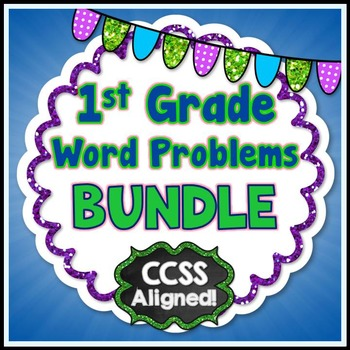DID YOU KNOW:
Seamlessly assign resources as digital activities

Learn how in 5 minutes with a tutorial resource. Try it Now

Learn More# Word Problems - 1st GradeGrade Levels
1st - 3rd, Homeschool
Subjects
Standards
Resource Type
Formats Included
• Zip
Pages
55 pages

### Description

Word Problems - 1st Grade

The steps in these math word (story) problems were created for the purpose of making sense of a problem, instead of using tricks and key words to solve a problem. Students must understand what the problem is asking them to do before solving it.

This bundle includes these three types of problems:
♦ the result is unknown (i.e. 9 + 5 = ?)
♦ the change is unknown (i.e. 9 + ? = 14)
♦ the start is unknown (i.e. ? + 5 = 14)

This collection of 44 math story problems focuses on the following Common Core Standards:
1.OA.1 Use addition and subtraction within 20 to solve word problems involving situations of adding to, taking from, putting together, taking apart, and comparing, with unknowns in all positions, e.g., by using objects, drawings, and equations with a symbol for the unknown number to represent the problem.
1.OA.2 Solve word problems that call for addition of three whole numbers whose sum is less than or equal to 20, e.g., by using objects, drawings, and equations with a symbol for the unknown number to represent the problem.
1.OA.3 Apply properties of operations as strategies to add and subtract.
1.OA.4 Understand subtraction as an unknown-addend problem.
1.OA.5 Relate counting to addition and subtraction (e.g., by counting on 2 to add 2).
1.OA.6 Add and subtract within 20, demonstrating fluency for addition and subtraction within 10.
1.OA.8 Determine the unknown whole number in an addition or subtraction equation relating to three whole numbers.

•••••••••••••••••••••••••••••••••••••••••••••••••••••••••••••••••••••••••••••••••••

If you are interested in purchasing every Common Core Math resource in my store for 1st grade (at a discounted price), please use the following link:
COMMON CORE MATH RESOURCES FOR 1ST GRADE

•••••••••••••••••••••••••••••••••••••••••••••••••••••••••••••••••••••••••••••••••••

Here are some other 1st grade products that you may be interested in:
NO PREP Math Printables for the Whole School Year!
Common Core Math Assessments for 1st Grade
October NO PREP Math Pack
Operations and Algebraic Thinking NO PREP Printables
Fall Fun Math Pack
Common Core Shape Activities
Place Value Match Game
Print n' Go Money Pack

•••••••••••••••••••••••••••••••••••••••••••••••••••••••••••••••••••••••••••••••••••

LET'S STAY CONNECTED!
Be the first to know about my new discounts, freebies and product launches:
• Look for the green star near the top of any page within MY STORE and click it to become a follower. You will then receive customized email updates about my store.
Total Pages
55 pages
Answer Key
Included
Teaching Duration
N/A
Report this Resource to TpT
Reported resources will be reviewed by our team. Report this resource to let us know if this resource violates TpT’s content guidelines.

### Standards

to see state-specific standards (only available in the US).
Determine the unknown whole number in an addition or subtraction equation relating three whole numbers. For example, determine the unknown number that makes the equation true in each of the equations 8 + ? = 11, 5 = ▯ - 3, 6 + 6 = ▯.
Add and subtract within 20, demonstrating fluency for addition and subtraction within 10. Use strategies such as counting on; making ten (e.g., 8 + 6 = 8 + 2 + 4 = 10 + 4 = 14); decomposing a number leading to a ten (e.g., 13 - 4 = 13 - 3 - 1 = 10 - 1 = 9); using the relationship between addition and subtraction (e.g., knowing that 8 + 4 = 12, one knows 12 - 8 = 4); and creating equivalent but easier or known sums (e.g., adding 6 + 7 by creating the known equivalent 6 + 6 + 1 = 12 + 1 = 13).
Relate counting to addition and subtraction (e.g., by counting on 2 to add 2).
Understand subtraction as an unknown-addend problem. For example, subtract 10 – 8 by finding the number that makes 10 when added to 8.
Apply properties of operations as strategies to add and subtract. If 8 + 3 = 11 is known, then 3 + 8 = 11 is also known. (Commutative property of addition.) To add 2 + 6 + 4, the second two numbers can be added to make a ten, so 2 + 6 + 4 = 2 + 10 = 12. (Associative property of addition.)

### Questions & Answers

Teachers Pay Teachers is an online marketplace where teachers buy and sell original educational materials.

More About Us